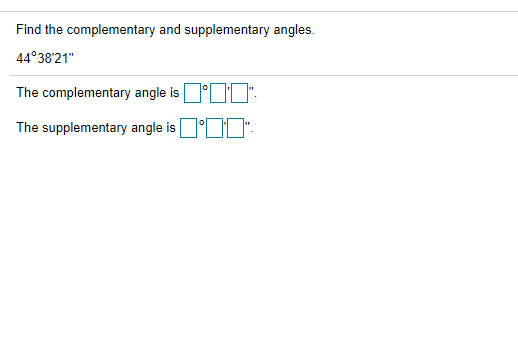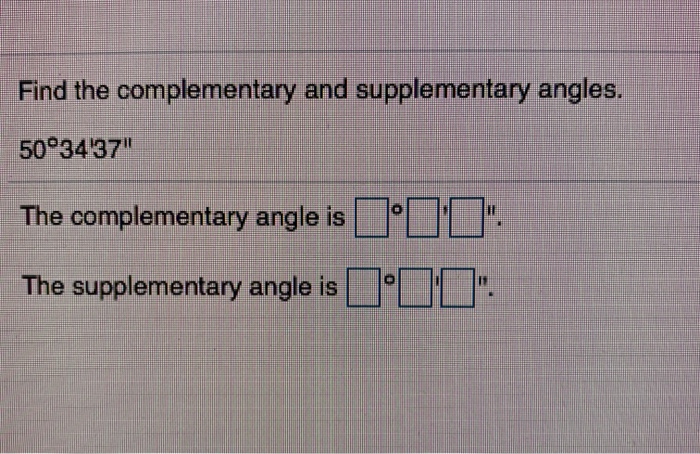Question
1.Two supplementary angles differ by 44 degrees.
2.Two complementary angles differ by 9 degrees.

1.
x + x - 44 = 180

2.
x + x - 9 = 90

#### Earn Coins

Coins can be redeemed for fabulous gifts.

Similar Homework Help Questions
• ### Find the complementary and supplementary angles. 44°38'21" The complementary angle is O'D'D": The supplementary angle is...Find the complementary and supplementary angles. 44°38'21" The complementary angle is O'D'D": The supplementary angle is O'DO".

• ### Find the measures of two supplementary angles if the difference of their measures is 56 degrees I know supplementary angles are the sum of the measure if two angles is 180 degrees but then what is the question asking

Find the measures of two supplementary angles if the difference of their measures is 56 degreesI know supplementary angles are the sum of the measure if two angles is 180 degrees but then what is the question asking?

• ### Find the complementary and supplementary angles. 50°3437" @ The complementary angle is The supplementary angle isFind the complementary and supplementary angles. 50°3437" @ The complementary angle is The supplementary angle is

• ### Two Angles are supplementary of their sum is 180°. One angle measures five times the measure...

Two Angles are supplementary of their sum is 180°. One angle measures five times the measure of a smaller angle. If x represents the measure of the smaller angle and these two angles are supplementary, find the measure of each angle. The smaller angle is _ degrees. The larger angle is _ degrees

• ### Two angles are supplementary

Two angles are supplementary. One angle is 4 degree less than 3 times the other. Find the measures of the angles.

• ### supplementary angles with measures 6x - 4 and 8x - 12 degrees

supplementary angles with measures 6x - 4 and 8x - 12 degrees

• ### supplementary angles where one angle measures 75 degrees

supplementary angles where one angle measures 75 degrees?

• ### supplementary angles with measures 10m + 7 and 7m + 3 degrees

supplementary angles with measures 10m + 7 and 7m + 3 degrees

• ### The difference between two supplementary angles is 50

The difference between two supplementary angles is 50. Find the two angles.

• ### Complementary angles are two angles whose measures add to 90 degrees

Complementary angles are two angles whose measures add to 90 degrees. The ratio of the measures of two complementary angles is 4:11. What are the measures of the angles?